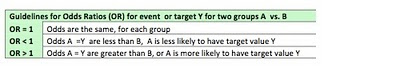## Thursday, March 10, 2011

### The Calculation and Interpretation of Odds Ratios

DATA

Assume some event with outcomes Y or N and two groups males vs females and the following historical data:

PROBABILITIES

Based on the data above, we can calculate the probabilities for Males and Females to have each outcome Y and N.

We can see that the probability that Males have event Y is greater than the probability for Females.

ODDS

From the probabilities we can calculate the odds of event Y for each group.

ODDS RATIOS

From the odds we can calculate the odds ratios for event Y, which give a comparison of the relative odds for each group M and F.

INTERPRETATION

From the basic probabilities above, we know that the probability of event Y is greater for males than females. The odds of event Y are also greater for males than females. These relationships are also reflected in the odds ratios. The odds of event Y for males is 3 times the odds of females. The odds of event Y for females are only .33 times the odds of males. In other words, the odds of event Y for males are greater and the odds of event Y for females is less.

This can also be seen from the formula for odds ratios. If the OR M vs F  = odds(M)/odds(F), we can see that if the odds (M) > odds(F), the odds ratio will be greater than 1. Alternatively, for OR  F vs M = odds(F)/odds(M), we can see that if the odds(F) < odds(M) then the ratio will be less than 1.  If the odds for both groups are equal, the odds ratio will be 1 exactly.

Three basic guidelines for interpreting odds ratios follow:Interpretation of the odds ratios above tells us that the odds of Y for females are less than the odds of males. It might be more informative if we could say how much less. To calculate this we need to go back to the raw odds calculations above.  In terms of percentages, the odds (Y) for females are [(.33-.11)/.33]*100 = -67%  or 67% less than the odds(Y)  for males.  This can be also be calculated more easily and directly from the odds ratio of F vs.M.

The odds(Y) for females are  [OR(F vs. M) – 1]*100 = [.33-1]*100 = -67% less than males.  A positive (vs. negative) result would imply an increased % of odds.

RELATION TO LOGISTIC REGRESSION

Odds ratios can be obtained from logistic regression by exponentiating the coefficient or beta for a given explanatory variable.  For categorical variables, the odds ratios are interpreted as above. For continuous variables, odds ratios are in terms of changes in odds as a result of a one-unit change in the variable.